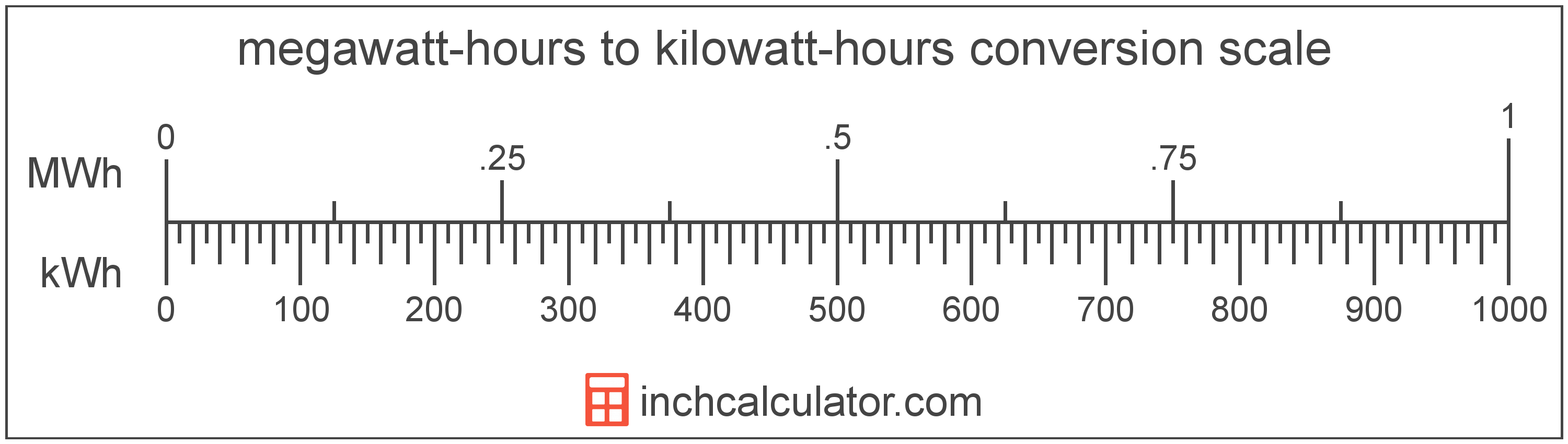# Kilowatt-Hours to Megawatt-Hours Converter

Enter the energy in kilowatt-hours below to get the value converted to megawatt-hours.

Results in Megawatt-Hours:1 kWh = 0.001 MWh

Do you want to convert megawatt-hours to kilowatt-hours?

## How to Convert Kilowatt-Hours to Megawatt-Hours

To convert a measurement in kilowatt-hours to a measurement in megawatt-hours, divide the energy by the following conversion ratio: 1,000 kilowatt-hours/megawatt-hour.

Since one megawatt-hour is equal to 1,000 kilowatt-hours, you can use this simple formula to convert:

megawatt-hours = kilowatt-hours ÷ 1,000

The energy in megawatt-hours is equal to the energy in kilowatt-hours divided by 1,000.

For example, here's how to convert 500 kilowatt-hours to megawatt-hours using the formula above.
megawatt-hours = (500 kWh ÷ 1,000) = 0.5 MWh## What Is a Kilowatt-Hour?

A kilowatt-hour is a measure of electrical energy equal to one kilowatt, or 1,000 watts, of power over a one hour period. Kilowatt-hours are a measure of electrical work performed over a period of time, and are often used as a way of measuring energy usage by electric companies.

Kilowatt-hours are usually abbreviated as kWh, although the formally adopted expression is kW·h. The abbreviation kW h is also sometimes used. For example, 1 kilowatt-hour can be written as 1 kWh, 1 kW·h, or 1 kW h.

In formal expressions, the centered dot (·) or space is used to separate units used to indicate multiplication in an expression and to avoid conflicting prefixes being misinterpreted as a unit symbol.

## What Is a Megawatt-Hour?

A megawatt-hour is a measure of electrical energy equal to one megawatt, or 1,000,000 watts, of power over a one hour period. Megawatt-hours are a measure of electrical work performed over a period of time, and are often used as a way of measuring energy usage by electric companies.

Megawatt-hours are usually abbreviated as MWh, although the formally adopted expression is MW·h. The abbreviation MW h is also sometimes used. For example, 1 megawatt-hour can be written as 1 MWh, 1 MW·h, or 1 MW h.

## Kilowatt-Hour to Megawatt-Hour Conversion Table

Table showing various kilowatt-hour measurements converted to megawatt-hours.
Kilowatt-hours Megawatt-hours
1 kWh 0.001 MWh
2 kWh 0.002 MWh
3 kWh 0.003 MWh
4 kWh 0.004 MWh
5 kWh 0.005 MWh
6 kWh 0.006 MWh
7 kWh 0.007 MWh
8 kWh 0.008 MWh
9 kWh 0.009 MWh
10 kWh 0.01 MWh
20 kWh 0.02 MWh
30 kWh 0.03 MWh
40 kWh 0.04 MWh
50 kWh 0.05 MWh
60 kWh 0.06 MWh
70 kWh 0.07 MWh
80 kWh 0.08 MWh
90 kWh 0.09 MWh
100 kWh 0.1 MWh
200 kWh 0.2 MWh
300 kWh 0.3 MWh
400 kWh 0.4 MWh
500 kWh 0.5 MWh
600 kWh 0.6 MWh
700 kWh 0.7 MWh
800 kWh 0.8 MWh
900 kWh 0.9 MWh
1,000 kWh 1 MWh

## References

1. Bureau International des Poids et Mesures, The International System of Units (SI), 9th edition, 2019, https://www.bipm.org/documents/20126/41483022/SI-Brochure-9-EN.pdf